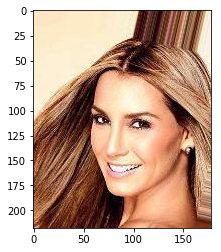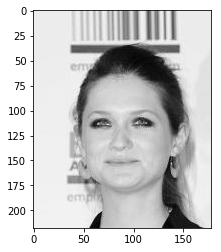Deep Learning Models -- A collection of various deep learning architectures, models, and tips for TensorFlow and PyTorch in Jupyter Notebooks.

In :
%load_ext watermark
%watermark -a 'Sebastian Raschka' -v -p torch

Sebastian Raschka

CPython 3.7.1
IPython 7.2.0

torch 1.0.0


# Model Zoo -- Using PyTorch Dataset Loading Utilities for Custom Datasets (Face Images from CelebA)¶

This notebook provides an example for how to load an image dataset, stored as individual JPEG files, using PyTorch's data loading utilities. For a more in-depth discussion, please see the official

In this example, we are using the CelebA face image dataset, which is available at http://mmlab.ie.cuhk.edu.hk/projects/CelebA.html. To execute the following examples, you need to download the "img_align_celeba.zip" (1.34 GB) file from the website and unzip in the current directory where this notebook is located. Similarly, download the attribute list "list_attr_celeba.txt" (25.48 MB) into this directory.

## Imports¶

In :
import pandas as pd
import numpy as np
import os

import torch
from torch.utils.data import Dataset
from torchvision import transforms

%matplotlib inline
import matplotlib.pyplot as plt
from PIL import Image


## Dataset¶

First, let's load the attribute list using Pandas. Here, we will be focussing on one attribute only, gender, which is stored in the "Male" column, where female face images are labeled as -1, and male images are labeled as 1. Further, we are binarizing these labels to 0's and 1's. The JPEG file names associated with each image are stored in the index of the following data frame:

In :
df = pd.read_csv('list_attr_celeba.txt', sep="\s+", skiprows=1, usecols=['Male'])

# Make 0 (female) & 1 (male) labels instead of -1 & 1
df.loc[df['Male'] == -1, 'Male'] = 0


Out:
Male
000001.jpg 0
000002.jpg 0
000003.jpg 1
000004.jpg 0
000005.jpg 0

## Preprocesing the Dataset¶

Next, we divide the dataset into 80% train and 20% testing data, and we save the file names and corresponding labels as separate text files:

In :
num_train_examples = int(df.shape*0.8)
df_train = df.iloc[:num_train_examples, :]
df_test = df.iloc[num_train_examples:, :]

df_train.to_csv('celeba_gender_attr_train.txt', sep=" ")
df_test.to_csv('celeba_gender_attr_test.txt', sep=" ")


Note that the images in CelebA are not ordered by gender, thus we do not have to worry about shuffling the dataset. Just as a quick sanity check, let's print the number of labels in the training and test datasets:

In :
print('Number of male and female images in training dataset:')
np.bincount(df_train['Male'].values)

Number of male and female images in training dataset:

Out:
array([94093, 67986])
In :
print('Number of male and female images in test dataset:')
np.bincount(df_test['Male'].values)

Number of male and female images in test dataset:

Out:
array([24072, 16448])

Let's also check that the text files load correctly using pandas read_csv function:

In :
pd.read_csv("celeba_gender_attr_train.txt", nrows=3, sep=" ", index_col=0)

Out:
Male
000001.jpg 0
000002.jpg 0
000003.jpg 1

Finally, let's open one of the images to make sure they look ok:

In :
img = Image.open('img_align_celeba/000001.jpg')
print(np.asarray(img, dtype=np.uint8).shape)
plt.imshow(img);

(218, 178, 3)## Implementing a Custom Dataset Class¶

Now, we implement a custom Dataset for reading the images. The __getitem__ method will

1. read a single image from disk based on an index (more on batching later)
2. perform a custom image transformation (if a transform argument is provided in the __init__ construtor)
3. return a single image and it's corresponding label
In :
class CelebaDataset(Dataset):

def __init__(self, txt_path, img_dir, transform=None):

df = pd.read_csv(txt_path, sep=" ", index_col=0)
self.img_dir = img_dir
self.txt_path = txt_path
self.img_names = df.index.values
self.y = df['Male'].values
self.transform = transform

def __getitem__(self, index):
img = Image.open(os.path.join(self.img_dir,
self.img_names[index]))

if self.transform is not None:
img = self.transform(img)

label = self.y[index]
return img, label

def __len__(self):
return self.y.shape


Now that we have created our custom Dataset class, let us add some custom transformations via the transforms utilities from torchvision, we

1. convert images to grayscale
2. normalize the images (here: dividing by 255)
3. converting the image arrays into PyTorch tensors

Then, we initialize a Dataset instance for the training images using the 'celeba_gender_attr_train.txt' label file (we omit the test set, but the same concepts apply).

Finally, we initialize a DataLoader that allows us to read from the dataset.

In :
# Note that transforms.ToTensor()
# already divides pixels by 255. internally

custom_transform = transforms.Compose([transforms.Grayscale(),
#transforms.Lambda(lambda x: x/255.),
transforms.ToTensor()])

img_dir='img_align_celeba/',
transform=custom_transform)

batch_size=128,
shuffle=True,
num_workers=4)


That's it, now we can iterate over an epoch using the train_loader as an iterator and use the features and labels from the training dataset for model training:

## Iterating Through the Custom Dataset¶

In :
device = torch.device("cuda:0" if torch.cuda.is_available() else "cpu")
torch.manual_seed(0)

num_epochs = 2
for epoch in range(num_epochs):

for batch_idx, (x, y) in enumerate(train_loader):

print('Epoch:', epoch+1, end='')
print(' | Batch index:', batch_idx, end='')
print(' | Batch size:', y.size())

x = x.to(device)
y = y.to(device)
break

Epoch: 1 | Batch index: 0 | Batch size: 128
Epoch: 2 | Batch index: 0 | Batch size: 128


Just to make sure that the batches are being loaded correctly, let's print out the dimensions of the last batch:

In :
x.shape

Out:
torch.Size([128, 1, 218, 178])

As we can see, each batch consists of 128 images, just as specified. However, one thing to keep in mind though is that PyTorch uses a different image layout (which is more efficient when working with CUDA); here, the image axes are "num_images x channels x height x width" (NCHW) instead of "num_images height x width x channels" (NHWC):

To visually check that the images that coming of the data loader are intact, let's swap the axes to NHWC and convert an image from a Torch Tensor to a NumPy array so that we can visualize the image via imshow:

In :
one_image = x.permute(1, 2, 0)
one_image.shape

Out:
torch.Size([218, 178, 1])
In :
# note that imshow also works fine with scaled
# images in [0, 1] range.

plt.imshow(one_image.to(torch.device('cpu')).squeeze(), cmap='gray');In :
%watermark -iv

pandas      0.23.4
numpy       1.15.4
torch       1.0.0
PIL.Image   5.3.0